# Exercises

1. Noting that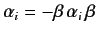, prove that the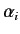and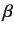matrices all have zero trace. Hence, deduce that each of these matrices has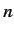eigenvalues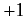, andeigenvalues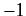, where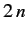is the dimension of the matrices.
2. Verify that the matrices (1125) and (1126) satisfy Equations (1117)-(1119).
3. Verify that the matrices (1123) and (1124) satisfy the anti-commutation relations (1122).
4. Verify that if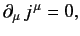where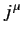is a 4-vector field, then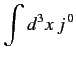is Lorentz invariant, where the integral is over all space, and it is assumed that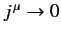as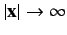.
5. Verify that (1168) is a solution of (1167).
6. Verify that the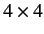matrices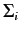, defined in (1189), satisfy the standard anti-commutation relations for Pauli matrices: i.e.,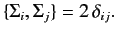Richard Fitzpatrick 2013-04-08